###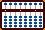Multiplication and Decimals

The following is an example where one of our group was having difficulty getting the unit number in the product answer to fall on a predetermined unit rod. Since his rod calculations were correct, the problem was solved in Step 2 of the first example.

##### Here's what I did with 93 x .026, I don't understand why my  answer is wrong.  93 = +2 .026 = -1 always add = +1 results start on rod +2 (rod E) put 93 on E and *F*

Hi Mr. K,

Following the rules for setting problems onto the soroban so that the unit number in the product falls naturally on a predetermined unit rod, your calculations are absolutely correct. Set 93 on E and *F*

PROBLEM: 1   93 x .026 = 2.418

93 x .026...........N =  2  - 1  + 1 = 2

NUMBERS ON THE SOROBAN

----A----B----C----D----E---*F*---G----H----I----J----K----
...+6...+5...+4...+3...+2...+1....0...-1...-2...-3...-4....

Step 1: Set the multiplicand 93 on EF and and the multiplier 26 on BC. (Fig.1)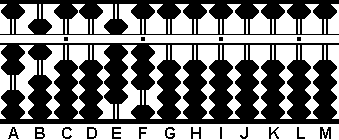Fig. 1 `Step 1` ```A B C D E F G H I J K L M . . . .   2 6 0 0 9 3 0 0 0 0 0 0 0 ```

Step 2:
Multiply the 3 on F by the 2 on A and add the product 06 to rods GH. (It's important to remember that when adding products to the soroban always think in terms of adding two numbers. For example 3 x 4 = 12 and 3 x 2 = 06)

2a: Multiply the 3 on F by the 6 on B and add the product 18 to HI. Clear the multiplicand 3 on F leaving 78 on rods HI. (Fig.2)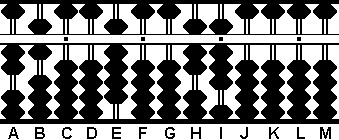Fig.2 `Step 2` ```A B C D E F G H I J K L M . . . .   2 6 0 0 9 3 0 0 0 0 0 0 0 + 0 6 Step 2 + 1 8 Step 2a 2 6 0 0 9 0 0 7 8 0 0 0 0```

Step 3:
Multiply the 9 on E by the 2 on A and add the product 18 to FG.

3a: Multiply the 9 on E by the 6 on B and add the product 54 to GH. Clear the multiplicand 9 on F leaving 2418 on rods FGHI. Because rod *F* is the unit rod the answer reads  2.418. (Fig.3)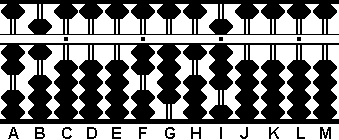Fig.3 `Step 3` ```A B C D E F G H I J K L M . . . .   2 6 0 0 9 0 0 7 8 0 0 0 0 + 1 8 Step 3 + 5 4 Step 3a 2 6 0 0 0 2 4 1 8 0 0 0 0 ```

PROBLEM: 2   6.0005 x .036 = 0.216018

6.0005 x .036...........N =  1  - 1  + 1 = 1

NUMBERS ON THE SOROBAN

----A----B----C----D----E---*F*---G----H----I----J----K----
...+6...+5...+4...+3...+2...+1....0...-1...-2...-3...-4....

Step 1:
Set the multiplicand 60005 on FGHIJ and and the multiplier 36 on CD. (Fig.4)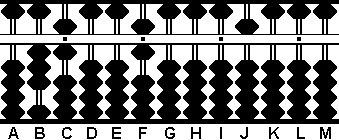Fig.4 `Step 1` ```A B C D E F G H I J K L M . . . .   0 3 6 0 0 6 0 0 0 5 0 0 0 ```

Step 2:
Multiply the 5 on J by the 3 on C and add the product 15 to rods KL.

2a: Multiply the 5 on J by the 6 on D and add the product 30 to LM. Clear the multiplicand 5 on J leaving 180 on rods KLM. (Fig.5)Fig.5 `Step 2` ```A B C D E F G H I J K L M . . . .   0 3 6 0 0 6 0 0 0 5 0 0 0 + 1 5 Step 2 + 3 0 Step 2a 0 3 6 0 0 6 0 0 0 0 1 8 0 ```

Step 3:
Multiply the 6 on F by the 3 on C and add the product 18 to GH.

3a: Multiply the 6 on F by the 6 on D and add the product 36 to HI. Clear the multiplicand 6 on F leaving 216018 on rods GHIJKL. Because rod F is the unit rod the answer reads 0.216018. (Fig.6)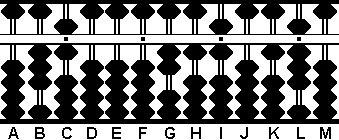Fig.6 `Step 3` ```A B C D E F G H I J K L M . . . .   0 3 6 0 0 6 0 0 0 0 1 8 0 + 1 8 Step 3 + 3 6 Step 3a 0 3 6 0 0 0 2 1 6 0 1 8 0```

- Totton